# Model not learning - Gives the same output for every example

Hi,
My data is in the shape of (11423, 2, 10, 34). I am dealing with sports data, where each instance is a matchup of two teams, with 10 players each, and 34 stats per player. I have data from 11423 matchups.

I want to use a 1D convolutional filter that is 10x1, to slide to the right across the 34 statistics for all 10 players on the two channels.
My target variable is the point differential for the game.
Here is what I have so far:

``````# Split up data - training set and testing set
X_train, X_test, y_train, y_test = train_test_split(matchup_features, targets, test_size=0.2)

print("X_train shape:", X_train.shape,
"\nX_test shape:", X_test.shape,
"\ny_test shape:", y_train.shape,
"\ny_train shape:", y_test.shape)
``````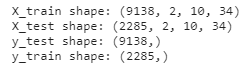``````class PlayerBoxScoreMatchupsDataset(Dataset):
def __init__(self, matchup_features, targets):
self.targets = targets
self.matchup_data = matchup_features

def __len__(self):
return len(self.matchup_data)

def __getitem__(self, index):
X = self.matchup_data[index]
y = self.targets[index]

return X, y

training_set = PlayerBoxScoreMatchupsDataset(X_train, y_train)
validation_set = PlayerBoxScoreMatchupsDataset(X_test, y_test)

print(training_set.shape, training_set)
````````````import torch.nn as nn
import torch.nn.functional as F

class Net(nn.Module):
def __init__(self):
super(Net, self).__init__()

self.conv1 = nn.Conv1d(2, 2, kernel_size=(10,1), stride=1)

self.fc1 = nn.Linear(68, 64)
self.fc2 = nn.Linear(64, 16)
self.fc3 = nn.Linear(16, 1)

self.dropout = nn.Dropout(0.25)

def forward(self, x):

x = F.relu(self.conv1(x))
#         print("shape after conv1:", x.shape)
x = x.reshape(-1, 68)
x = self.dropout(x)
x = F.relu(self.fc1(x))
x = self.dropout(x)
x = F.relu(self.fc2(x))
x = self.dropout(x)
x = self.fc3(x)

return x

net = Net()
print(net)
``````
``````import torch.optim as optim

net = Net()
loss_function = nn.MSELoss()

epochs = 30

for e in range(epochs):
train_loss = 0
val_loss = 0
net.train()
output = net(data.float())
if e == 29:
print("first output of batch:", output)
print("first target of batch:", target)
loss = loss_function(output, target.float())

loss.backward()

optimizer.step()
train_loss += loss.item() * data.size(0)

net.eval()
output = net(data.float())
loss = loss_function(output, target.float())

val_loss += loss.item()*data.size(0)

print('Epoch: {} \tTraining Loss: {:.4f} \tValidation Loss: {:.4f}'.format(
e, train_loss, val_loss))
``````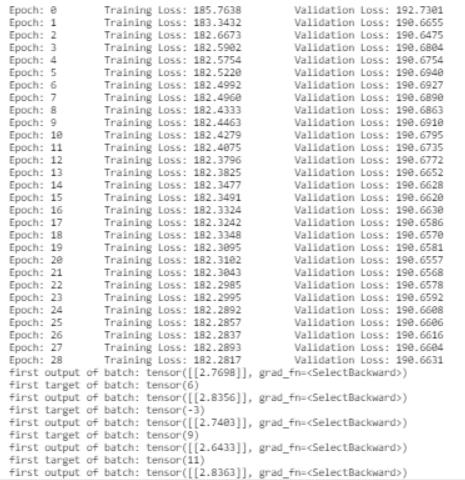As you can see, the model barely learns, and worse, it predicts about the same thing for every example (even in the training set! - about 2.7, even if that is no where near the actual target).

I’ve tried adjusting the learning rate, and it did not change anything. I’m thinking something may be wrong with the
`conv1d(2, 2, kernel_size=(10,1), stride=1)` part because that part is most hazy to me.
What mistake am I making? Thanks for your help!

maybe try and add some batch normalization:
https://pytorch.org/docs/stable/generated/torch.nn.BatchNorm1d.html

Hi, thanks for the response.
I tried adding batch normalization as such:

``````import torch.nn as nn
import torch.nn.functional as F

class Net(nn.Module):
def __init__(self):
super(Net, self).__init__()

self.conv1 = nn.Conv1d(2, 2, kernel_size=(10,1), stride=1)
self.conv1_bn = nn.BatchNorm2d(2)

self.fc1 = nn.Linear(68, 64)
self.fc1_bn = nn.BatchNorm1d(64)
self.fc2 = nn.Linear(64, 16)
self.fc2_bn = nn.BatchNorm1d(16)
self.fc3 = nn.Linear(16, 1)

self.dropout = nn.Dropout(0.25)

def forward(self, x):

x = self.conv1_bn(F.relu(self.conv1(x)))
#         print("shape after conv1:", x.shape)
x = x.reshape(-1, 68)
x = self.dropout(x)
x = self.fc1_bn(F.relu(self.fc1(x)))
x = self.dropout(x)
x = self.fc2_bn(F.relu(self.fc2(x)))
x = self.dropout(x)
x = self.fc3(x)

return x

net = Net()
print(net)
``````

However I still ended up with similar results: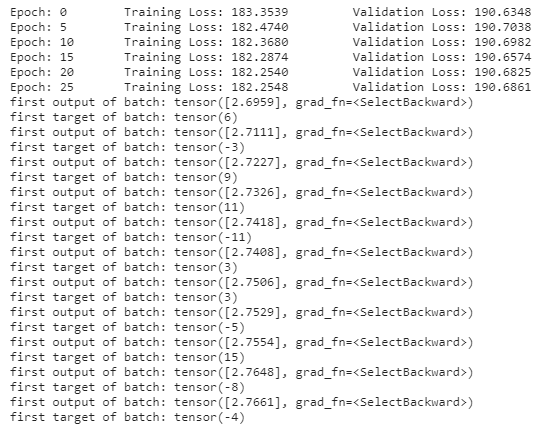Any other ideas?

Hi Jordan,
Without seeing the data it’s difficult to say what might work. However, you may try adding a non-linearity (e.g. Sigmoid or ReLU) after your Linear transformations.

Thank you for the response. I tried adding different activation functions and got the similar results.
One other thing I tried which may be telling are:

1. My network can reach 0 loss on a single training example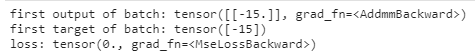However as soon as I try to train a batch of two inputs, it predicts the same output: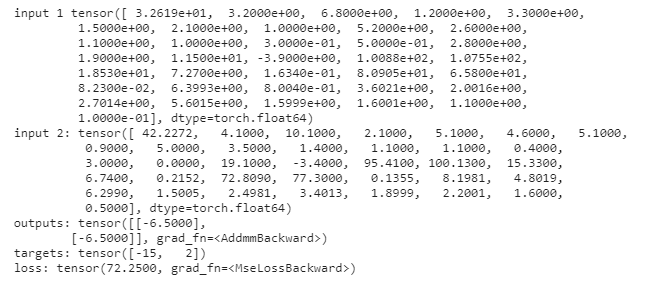As you can see the inputs are different but they both predict -6.5, when the actual targets are -15 and 2.

Any idea of what this means and/or how I can try to debug it? Thank you!